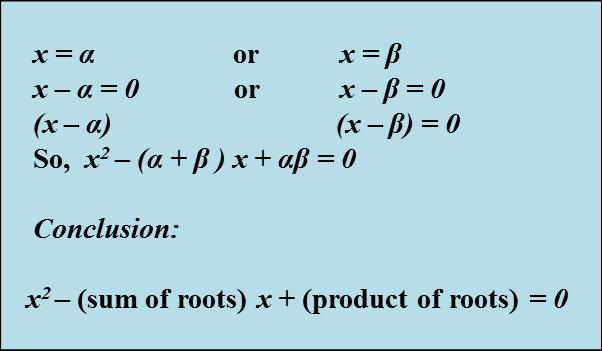\

# 2.4 Forming Quadratic Equations from Given Roots

2.4 Forming Quadratic Equations from Given Roots

Let α and β be the roots of the equation ax2 + bx + c = 0, this meansExample:
Find the quadratic equation from the respective roots below:
(a) 3, 1
(b) 2, ¼
(c) , ¼
(d) 3m,2m

Solution: